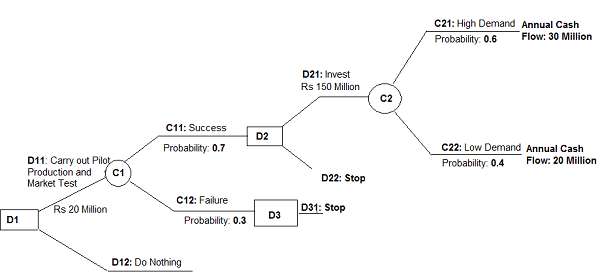# Decision Tree Analysis

Definition: The Decision Tree Analysis is a schematic representation of several decisions followed by different chances of the occurrence. Simply, a tree-shaped graphical representation of decisions related to the investments and the chance points that help to investigate the possible outcomes is called as a decision tree analysis.

The decision tree shows Decision Points, represented by squares, are the alternative actions along with the investment outlays, that can be undertaken for the experimentation. These decisions are followed by the chance points, represented by circles, are the uncertain points, where the outcomes are dependent on the chance process. Thus, the probability of occurrence is assigned to each chance point.Once the decision tree is described precisely, and the data about outcomes along with their probabilities is gathered, the decision alternatives can be evaluated as follows:

1. Start from the extreme right-hand end of the tree and start calculating NPV for each chance points as you proceed leftward.
2. Once the NPVs are calculated for each chance point, evaluate the alternatives at the final stage decision points in terms of their NPV.
3. Select the alternative which has the highest NPV and cut the branch of inferior decision alternative. Assign value to each decision point equivalent to the NPV of the alternative selected.
4. Again, repeat the process, proceed leftward, recalculate NPV for each chance point, select the decision alternative which has the highest NPV value and then cut the branch of the inferior decision alternative. Assign the value to each point equivalent to the NPV of selected alternative and repeat this process again and again until a final decision point is reached.

Thus, decision tree analysis helps the decision maker to take all the possible outcomes into the consideration before reaching a final investment decision.